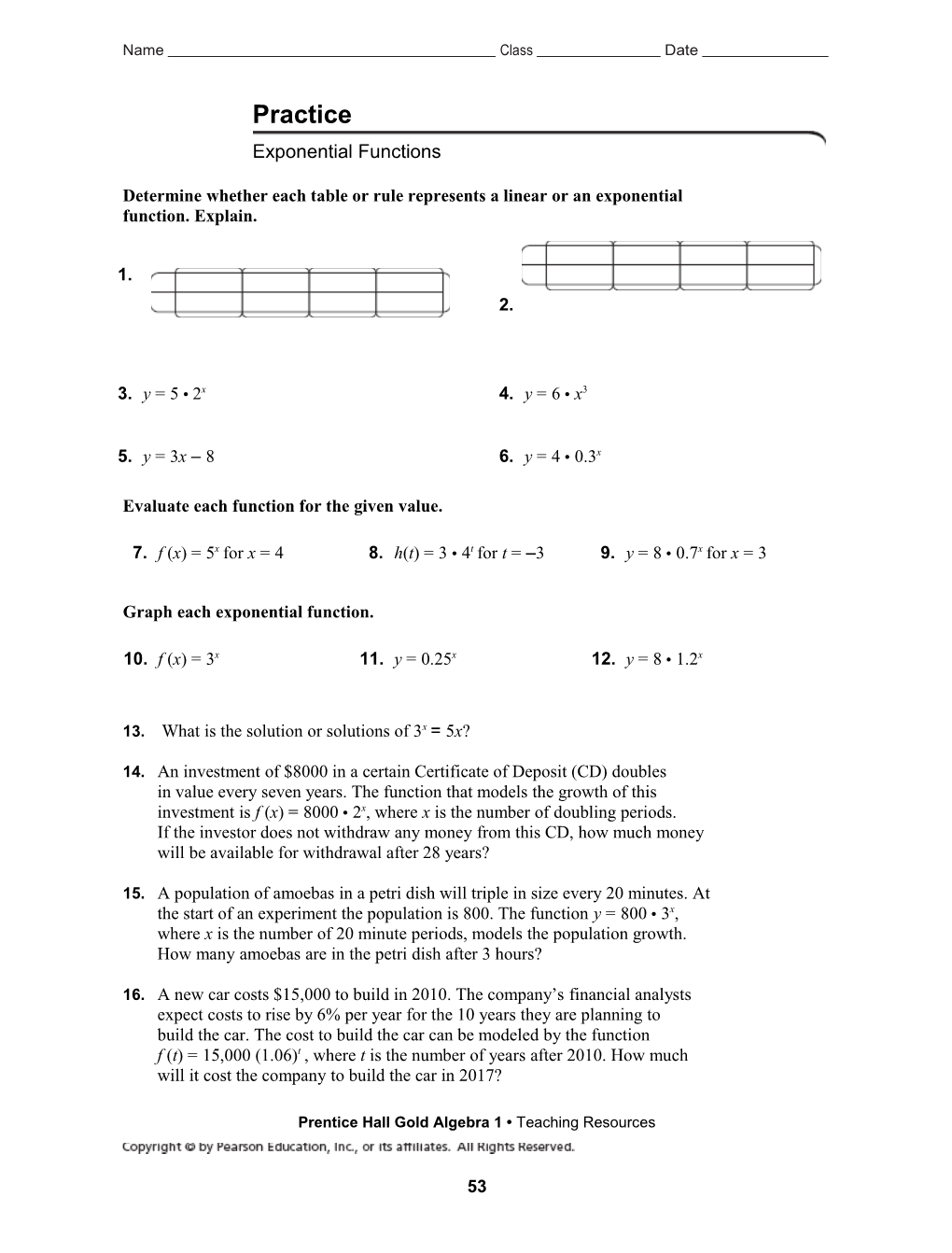# Evaluate Each Function for the Given ValueName Class Date

Practice

Exponential Functions

Determine whether each table or rule represents a linear or an exponential
function. Explain.

1. / 2.
3.y =5 • 2x / 4.y =6 •x3
5.y =3x – 8 / 6.y =4 •0.3x

Evaluate each function for the given value.

7.f (x) =5xfor x =4 / 8.h(t) =3 •4tfor t = –3 / 9.y =8 •0.7xfor x =3

Graph each exponential function.

10.f (x) =3x / 11.y =0.25x / 12.y =8 •1.2x

13.What is the solution or solutions of 3x= 5x?

14.An investment of \$8000 in a certain Certificate of Deposit (CD) doubles
in value every seven years. The function that models the growth of this
investment is f (x) =8000 •2x, where x is the number of doubling periods.
If the investor does not withdraw any money from this CD, how much money
will be available for withdrawal after 28 years?

15.A population of amoebas in a petri dish will triple in size every 20 minutes. At
the start of an experiment the population is 800. The function y =800 •3x,
where x is the number of 20 minute periods, models the population growth.
How many amoebas are in the petri dish after 3 hours?

16.A new car costs \$15,000 to build in 2010. The company’s financial analysts
expect costs to rise by 6% per year for the 10 years they are planning to
build the car. The cost to build the car can be modeled by the function
f (t) =15,000 (1.06)t, where t is the number of years after 2010. How much
will it cost the company to build the car in 2017?

Prentice Hall Gold Algebra 1 • Teaching Resources

53

Name Class Date

Practice (continued)

Exponential Functions

Evaluate each function over the domain {–2, –1, 0, 1, 2, 3}. As the values of the

domain increase, do the values of the range increase or decrease?

17.f (x) =3x / 18.y =4.2x
19.m(x) =0.3x / 20.g(t) =4 •3x
21.y =50 •0.1x / 22.f(x) =2 •4x

Which function has the greater value for the given value of x?

23.y =5xor y =x5for x =2 / 24.y =300 •x3or y =100 •3xfor x =4

Solve each equation.

25.3x=81 / 26.5 •2x=40
27.4x+ 4 =68 / 28.3 •2x– 16 =80

29.Reasoning The function that models the growth of a \$1000 investment that
earns 7% per year is f(x) =1000(1.07)x. How do you think you would write
a function that models the growth of \$1500 that earns 8% per year? Use that
function to determine how much money a person would have after 5 years if
she invested \$1500 in an account earning 8% per year.

30.Writing Discuss the differences between exponential functions with a base
of 2 and 3, y =2xand y =3x, and quadratic and cubic functions y =x2and
y =x3. Focus on the shapes of the different graphs and rates of growth.

31.Open-Ended Find the value of each of the functions a) f(x) =2x2and
b) f(x) =2 •2xfor x =5. Write another quadratic function and another
exponential function with a base of two whose values at x =5 are between
the values you found for functions a and b.

Prentice Hall Gold Algebra 1 • Teaching Resources

54